# myCBSEguide App

Install myCBSEguide mobile app for FREE sample papers, Test Papers, Revision Notes, Previous year question papers, NCERT solutions and MCQ tests. Refer myCBSEguide App to your friends and Earn upto Rs.500/-.Download NCERT solutions for Vector Algebra as PDF.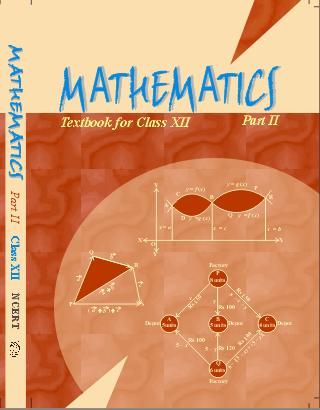## NCERT Solutions class 12 Maths Vector Algebra

1. Represent graphically a displacement of 40 km,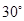east of north.

Ans. Displacement 40 km,East of North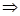Displacement vector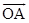(say) such that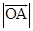= 40 (given) and vectormakes an anglewith North in East-North quadrant.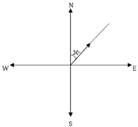#### 2. Check the following measures as scalars and vectors:

(i) 10 kg

(ii) 2 meters north-west

(iii)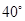(iv) 40 Watt

(v)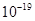coulomb

(vi) 20 m/sec2

Ans. (i) 10 kg is a measure of mass, it has no direction, it is magnitude only and therefore it is a scalar.

(ii) 2 meters North-West us a measure of velocity. It has magnitude and direction both and hence it is a vector.

(iii)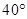is a measure of angle. It has no direction, it has magnitude only. Therefore it is a scalar.

(iv) 40 Watt is a measure of power. It has no direction, only magnitude and therefore, it is a scalar.

(v)coulomb is a measure of electric charge and it has magnitude only, therefore, it is a scalar.

(vi) 20 m/sec2 is a measure of acceleration. It is a measure of rate of change of velocity, therefore, it is a vector.

#### 3. Classify the following as scalar and vector quantities:

(i) time period

(ii) distance

(iii) force

(iv) velocity

(v) work done

Ans. (i) Time-scalar

(ii) Distance-scalar

(iii) Force-vector

(iv) Velocity-vector

(v) Work done-scalar

#### 4. In the adjoining figure, (a square) identify the following vectors: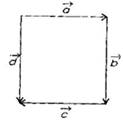(i) Coinitial

(ii) Equal

(iii) Collinear but not equal

Ans. (i)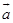and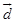have same initial point and therefore coinitial vectors.

(ii)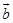andhave same direction and same magnitude. Thereforeandare equal vectors.

(iii)and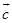have parallel support, so that they are collinear. Since they have opposite directions, they are not equal. Henceandare collinear but not equal.

#### 5. Answer the following as true or false:

(i)and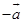are collinear.

(ii) Two collinear vectors are always equal in magnitude.

(iii) Two vectors having same magnitude are collinear.

(iv) Two collinear vectors having the same magnitude are equal.

Ans. (i) True.

(ii) False. [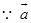and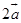are collinear vectors but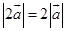]

(iii) False. [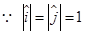but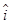and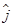are vectors along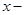axis (OX) and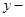axis (OY) respectively]

(iv) False. [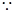Vectorsand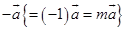are collinear vectors and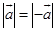but we know that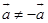because their directions are opposite.]

## NCERT Solutions class 12 Maths Exercise 10.1

NCERT Solutions Class 12 Maths PDF (Download) Free from myCBSEguide app and myCBSEguide website. Ncert solution class 12 Maths includes text book solutions from both part 1 and part 2. NCERT Solutions for CBSE Class 12 Maths have total 13 chapters. 12 Maths NCERT Solutions in PDF for free Download on our website. Ncert Maths class 12 solutions PDF and Maths ncert class 12 PDF solutions with latest modifications and as per the latest CBSE syllabus are only available in myCBSEguide

## CBSE App for Students

To download NCERT Solutions for class 12 Physics, Chemistry, Biology, History, Political Science, Economics, Geography, Computer Science, Home Science, Accountancy, Business Studies and Home Science; do check myCBSEguide app or website. myCBSEguide provides sample papers with solution, test papers for chapter-wise practice, NCERT solutions, NCERT Exemplar solutions, quick revision notes for ready reference, CBSE guess papers and CBSE important question papers. Sample Paper all are made available through the best app for CBSE students and myCBSEguide website.